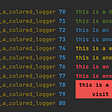# As predicted delayed outputs settle in NarX

I applied my code to data simplenarx_dataset. To do this I performed the following steps:

1 — I have done autocorrelation and cross correlation peaks to see that gives us more information. ID = 1, FD = 1

2 — I have found H, where H = 5

3 — I have created the network and have evaluated the details. Although the purpose of this post is not to evaluate the details but understand why you see a delayed response when performing closeloop, but public details and code In case of emergency there is some other error: My code is as follows(I used 80 data for training the network and 20 to check with closeloop):

p=p’;

t=t’;

p1=p(1:1,1:80);

p2=p(1:1,81:end);

t1=t(1,1:80);

t2=t(1,81:end);

inputSeries = tonndata(p1,true,false);

targetSeries = tonndata(t1,true,false);

inputDelays = 1:1;

feedbackDelays = 1:1;

hiddenLayerSize = 5;

net = narxnet(inputDelays,feedbackDelays,hiddenLayerSize);

[inputs,inputStates,layerStates,targets] = preparets(net,inputSeries,{},targetSeries);

net.divideFcn=’divideblock’;

net.divideParam.trainRatio=0.70;

net.divideParam.valRatio=0.15;

net.divideParam.testRatio=0.15;

[I N]=size(p1);

[O N]=size(t1);

N=N-1;

Neq=N*O;

ID=1;

FD=1;

Nw = (ID*I+FD*O+1)*hiddenLayerSize+(hiddenLayerSize+1)*O;

Ntrneq = N -2*round(0.15*N);

Ndof=Ntrneq-Nw;

ttotal=t1(1,1:N);

MSE00=mean(var(ttotal,1));

MSE00a=mean(var(ttotal,0));

t3=t(1,1:N);

[trainInd,valInd,testInd] = divideblock(t3,0.7,0.15,0.15);

MSEtrn00=mean(var(trainInd,1));

MSEtrn00a=mean(var(trainInd,0));

MSEval00=mean(var(valInd,1));

MSEtst00=mean(var(testInd,1));

net.trainParam.goal = 0.01*Ndof*MSEtrn00a/Ntrneq;

[net,tr,Ys,Es,Xf,Af] = train(net,inputs,targets,inputStates,layerStates);

outputs = net(inputs,inputStates,layerStates);

errors = gsubtract(targets,outputs);

MSE = perform(net,targets,outputs);

MSEa=Neq*MSE/(Neq-Nw);

R2=1-MSE/MSE00;

R2a=1-MSEa/MSE00a;

MSEtrn=tr.perf(end);

MSEval=tr.vperf(end);

MSEtst=tr.tperf(end);

R2trn=1-MSEtrn/MSEtrn00;

R2trna=1-MSEtrn/MSEtrn00a;

R2val=1-MSEval/MSEval00;

R2tst=1-MSEtst/MSEtst00;

and my results are:

ID=1

FD=1

H=5

N=79

Ndof=34

Neq=79

Ntrneq=55

Nw=21

O=1

I=1

R2=0.8036

R2a=0.7347

R2trn=0.8763

R2trna=0.8786

R2val=0.7862

R2tst=0.7541

As I mentioned earlier, I will not focus much on the accuracy in the answer but later will. The code I applied for closeloop was:

netc = closeloop(net);

netc.name = [net.name ‘ — Closed Loop’];

view(netc)

NumberOfPredictions = 15;

s=cell2mat(inputSeries);

t4=cell2mat(targetSeries);

a=s(1:1,79:80);

b=p2(1:1,1:15);

newInputSeries=[a b];

c=t4(1,80);

d=nan(1,16);

newTargetSet=[c d];

newInputSeries=tonndata(newInputSeries,true,false);

newTargetSet=tonndata(newTargetSet,true,false);

[xc,xic,aic,tc] = preparets(netc,newInputSeries,{},newTargetSet);

yPredicted = sim(netc,xc,xic,aic);

w=cell2mat(yPredicted);

plot(cell2mat(yPredicted),’DisplayName’,’cell2mat(yPredicted)’,’YdataS

ource’,’cell2mat(yPredicted)’);figure(gcf)

plot(t2,’r’,’DisplayName’,’targetsComprobacion’)

hold on

title({‘ITERACCIONES’})

legend(‘show’)

hold off

and the result was the chart that you have indicated the link below where you will see it:

http://www.subirimagenes.com/otros-simenarx-8376264.html

In this picture we see the blue line (line outputs predicted) lags behind the red line (real targets). I’d like to know how I can do to get that blue line is in front of the red line, that is one step get out early. As I said, in this post I want to focus on why this happens and how I can fix it.

NOTE:-

Matlabsolutions.com provide latest MatLab Homework Help,MatLab Assignment Help for students, engineers and researchers in Multiple Branches like ECE, EEE, CSE, Mechanical, Civil with 100% output.Matlab Code for B.E, B.Tech,M.E,M.Tech, Ph.D. Scholars with 100% privacy guaranteed. Get MATLAB projects with source code for your learning and research.

% 1. Selected ending semicolons can be removed to aid debugging

`[P, T ] = simplenarx_dataset; whos p= cell2mat(P); t = cell2mat(T); ID = 1:1 FD = 1:1 H = 5 NID= length(ID) NFD=length(FD) Nw = (NID*I+NFD*O+1)*H+(H+1)*O`

% 2. Use NID and NFD for Nw in case delays are not single

% 3. No need to use tonndata because the simplenarx_data set is instantly ready for preparets.

% 4. No need for (p1,t1) and (p2,t2). Delete both.

% 5. Input delays are suboptimal. Did you try to find the significant lags of the target/input cross-correlation function?

% 6. Feedback delays are suboptimal. Did you try to find the significant lags of the target autocorrelation function?

% 7. H is suboptimal. Was it chosen using the suboptimal delays? If so, please explain how.

`rng(0) net = narxnet(ID,FD,H); [inputs,inputStates,layerStates,targets] = preparets(net,P,{},T); whos P T inputs inputStates layerStates targets`

%8. N=N-1: DELETE. NOT A GOOD IDEA TO USE A VARIABLE OR PARAMETER NAME ON BOTH SIDES OF AN EQUATION. BESIDES, PREPARETS OUTPUTS THE CORRECT DIMENSIONS

`[ I N ] = size(inputs) [ O N ] = size(targets)`

% 9. No need for ttotal it should be the same as targets. % No need for Neq, MSE00,MSE00a and t4. Delete

`net.divideFcn='divideblock'; [trainInd,valInd,testInd] = divideblock(N,0.7,0.15,0.15);  ttrn = targets(trainInd); tval = targets(valInd); ttest = targets(testInd); Ntst = length(ttrn)  Nval = length(valInd) Ntst = length(testInd) Ntrneq = prod(size(ttrn))     % Ntrn*O Ndof = Ntrneq-Nw`

%Naive Constant Output Model

--

--

--

## More from Technical Source

Simple! That is me, a simple person. I am passionate about knowledge and reading. That’s why I have decided to write and share a bit of my life and thoughts to.

Love podcasts or audiobooks? Learn on the go with our new app.

## Use Vagrant to Improve Development Environment Setup Time## How to edit PYTHONPATH on Windows## Maneki-Meow on Conflux Testnet## Colored Logs for Python## Reading 08: say fork 10 times fast## Technical Source

Simple! That is me, a simple person. I am passionate about knowledge and reading. That’s why I have decided to write and share a bit of my life and thoughts to.

## Predicting rat’s location with ML models## Identifying Differences among Individuals’ Functional Connectivity Patterns## Time series forecasting of bitcoin prices.